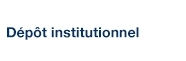# Quantum Population Dynamics: A Broad View from an Exploration beyond the Standard Model

Blanchet, Jean-Pierre (2015). « Quantum Population Dynamics: A Broad View from an Exploration beyond the Standard Model ». Quantum Physics Letters, 4(2), pp. 31-50.

Fichier(s) associé(s) à ce document :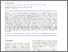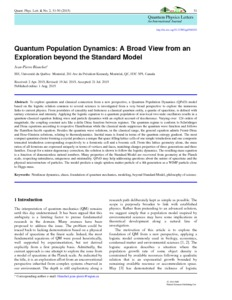Prévisualisation
PDF
Télécharger (1MB)

## Résumé

To explore quantum and classical connection from a new perspective, a Quantum Population Dynamics (QPoD) model based on the logistic relation common to several sciences is investigated from a very broad perspective to explore the numerous links to current physics. From postulates of causality and finiteness a classical quantum entity, a quanta of spacetime, is defined with unitary extension and intensity. Applying the logistic equation to a quantum population of non-local two-state oscillators results in a quantum-classical equation linking wave and particle dynamics with an explicit account of decoherence. Varying over 124 orders of magnitude, the coupling constant acts like a delta Dirac function between regimes. The quantum regime is conform to Schrödinger and Dirac equations according to respective Hamiltonian while the classical mode suppresses the quantum wave function and follows the Hamilton-Jacobi equation. Besides the quantum wave solutions, in the classical range, the general equation admits Fermi-Dirac and Bose-Einstein solutions, relating to thermodynamics. Inertial mass is found in terms of the quantum entropy gradient. The most compact quantum cluster forming a crystal produces a unique flat space filling lattice cells of one simple tetrahedron and one composite truncated tetrahedron corresponding respectively to a fermionic cell and a bosonic cell. From this lattice geometry alone, the mass ratios of all fermions are expressed uniquely in terms of vertices and faces, matching charges properties of three generations and three families. Except for a minor degeneracy correction, the solution is shown to follow the logistic dynamics. The resulting mass equation is a function of dimensionless natural numbers. Many properties of the Standard Model are recovered from geometry at the Planck scale, respecting naturalness, uniqueness and minimality. QPoD may help addressing questions about the nature of spacetime and the physical microstructure of particles. The model predicts a single spinless matter particle of a 4th generation as a WIMP particle close to Higgs mass.

Type: Article de revue scientifique Nonlinear dynamics, chaos, foundation of quantum mechanics, modeling, beyond Standard Model, philosophy of science Centres institutionnels > Centre pour l'étude et la simulation du climat à l'échelle régionale (ESCER) Jean-Pierre Blanchet 22 janv. 2021 13:44 22 janv. 2021 13:44 http://archipel.uqam.ca/id/eprint/13892

## Statistiques

Voir les statistiques sur cinq ans...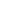RECHERCHER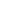PARCOURIR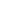LIBRE ACCÈS